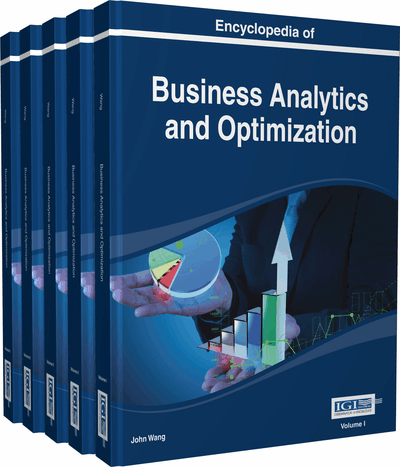# OLAP over Uncertain and Imprecise Data Streams

Alfredo Cuzzocrea (ICAR-CNR, Italy & University of Calabria, Italy)
DOI: 10.4018/978-1-4666-5202-6.ch149

Top

## Introduction

A critical issue in representing, querying and mining data streams consists of the fact that they are intrinsically multi-level and multidimensional in nature (Cai et al., 2004; Han et al., 2005), hence they require to be analyzed by means of multi-level and multi-resolution (analysis) models accordingly. Furthermore, it is a matter of fact to note that enormous data flows generated by a collection of stream sources naturally require to be processed by means of advanced analysis/mining models, beyond traditional solutions provided by primitive SQL-based DBMS interfaces, and very often high-performance computational infrastructures, like Data Grids, are advocated to provide the necessary support to this end (e.g., (Cuzzocrea et al., 2004a; Cuzzocrea et al., 2004b; Cuzzocrea et al., 2005)), also exploiting fortunate data compression paradigms (e.g., (Cuzzocrea, 2005; Cuzzocrea, 2006a; Cuzzocrea, 2006b; Cuzzocrea and Wang, 2007; Cuzzocrea et al., 2007; Cuzzocrea et al., 2009b; Cuzzocrea & Serafino, 2009)) or data fragmentation paradigms (e.g., (Bonifati & Cuzzocrea, 2007)). Conventional analysis/mining tools (e.g., DBMS-inspired) cannot carefully take into consideration these kinds of multidimensionality and correlation of real-life data streams, as stated in (Cai et al., 2004; Han et al., 2005). From this, it follows that, if one tries to process multidimensional and correlated data streams by means of such tools, rough errors are obtained in practice, thus seriously affecting the quality of decision making processes that found on analytical results mined from streaming data.

Modern data stream applications and systems are also more and more characterized by the presence of uncertainty and imprecision that make the problem of dealing with uncertain and imprecise data streams a leading research challenge. This issue has recently attracted a great deal of attention from both the academic and industrial research community, as confirmed by several research efforts done in this context (Cormode & Garofalakis, 2007; Jayram et al., 2007; Aggarwal & Yu, 2008; Cormode et al., 2008; Jin et al., 2008; Zhang et al., 2008; Etuk et al., 2013).

Uncertain and imprecise data streams arise in a plethora of actual application scenarios ranging from environmental sensor networks to logistic networks and telecommunication systems, and so forth. Consider, for instance, the simplest case of a sensor network monitoring the temperature T of a given geographic area W. Here, being T monitoring a natural, real-life measure, it is likely to retrieve an estimate of T, denoted by, with a given confidence interval, denoted by [,], such that<, having a certain probability pT, such that 0 ≤ pT 1, rather than to obtain the exact value of T, denoted by. The semantics of this confidence-interval-based model states that the (estimated) value of T,, ranges betweenandwith probability pT . Also, a law describing the probability distribution according to which possible values of T vary over the interval [,] is assumed. Without loss of generality, the uniform distribution is very often taken as reference. The uniform distribution states that (possible) values in [,], have all the same probability to be the exact value of T,, effectively. Despite the popularity of the normal distribution, the confidence-interval-based model above is prone to incorporate any other kind of probability distribution (Papoulis, 1994).

## Key Terms in this Chapter

Probabilistic Estimators Theory: Branch of statistics focused on estimating the values of parameters based on measured data that has a random component.

Data Cube: A multidimensional dataset used to explore and analyze business data from many different perspectives.

OLAP: On-Line Analytical Processing, or OLAP, designate a set of software techniques for interactive analysis of large amounts of multidimensional data from multiple perspectives.

Data Stream: Continuous and transient flow of data (usually coming from sensors, web applications, or telecommunication networks) processed by advanced analysis techniques.

Possible-World Semantics: Semantics for evaluating queries over uncertain and imprecise probabilistic databases.

Uncertain and Imprecise Data Stream: Data stream in which the data obtained are inherently inaccurate, due to their continuous-changing nature.

Probability Distribution Function: In probability and statistics, it is the function that describe the probability distribution of the possible values of a random variable.

Business Intelligence: A set of theories, methodologies, architectures, and technologies that transform raw data into meaningful and useful information and knowledge for business purposes, by handling large amounts of both structured and unstructured data.

## Complete Chapter List

Search this Book:
Reset